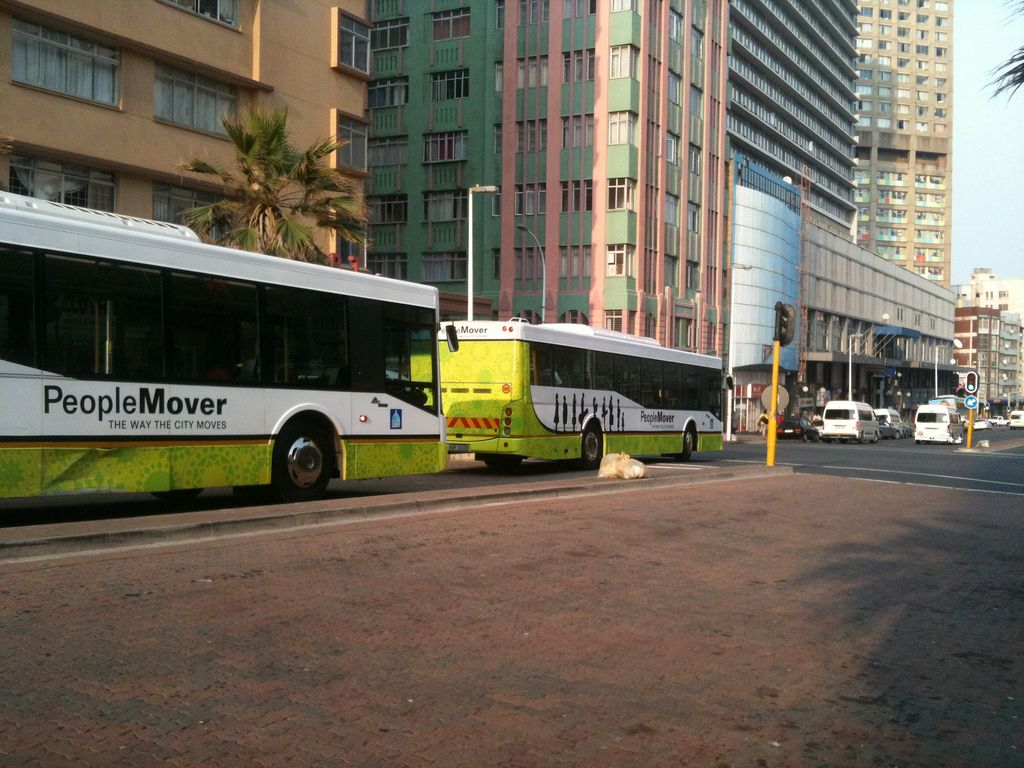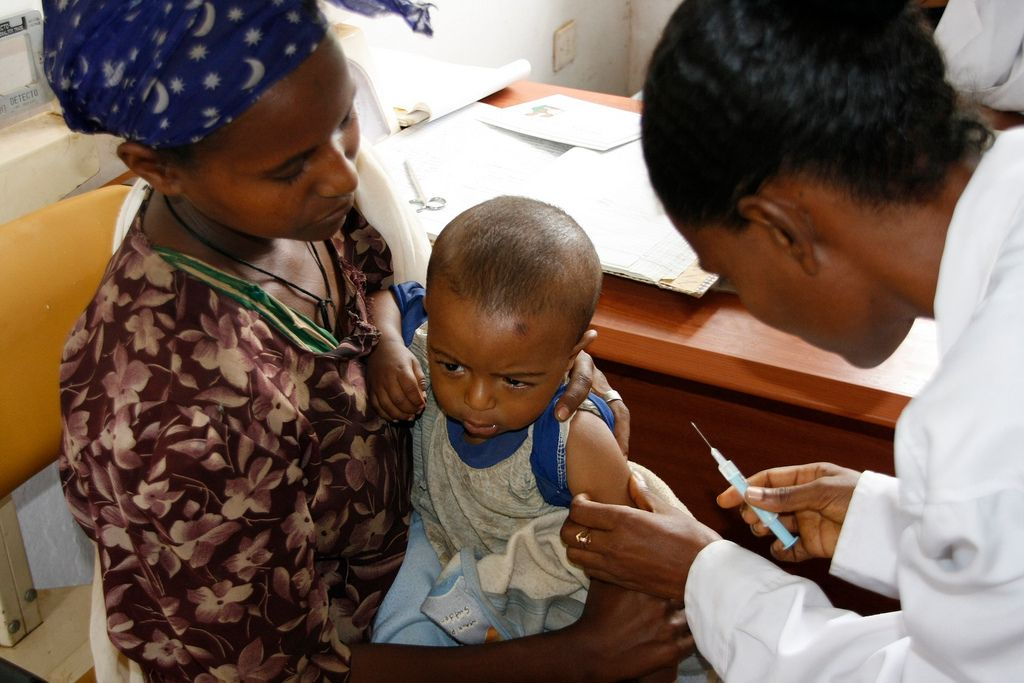Home Practice
For learners and parents For teachers and schools
Textbooks
Full catalogue
Pricing SupportLog in

We think you are located in United States. Is this correct?

# 8.4 The difference between budgets and statements

## 8.4 The difference between budgets and statements (EMG5K)

A statement is a record of actual expenditure, rather than planned expenditure. We usually compare the actual expenditure to planned expenses at this stage.

## Understanding a statement

Exercise 8.5

Consider the previous activity, where Douglas planned to travel to Durban. He eventually decided to travel to Durban using bus Option 1. He kept all the receipts and till slips so that he could write a statement to see how much money he actually spent. Read the following summary of Douglas's bus trip:

When Douglas arrived at the bus station to buy the ticket, he finds that the advertised price did not include VAT, and he needs to add $$\text{14}\%$$ to the cost. To add to his problems, the bus breaks down and Douglas needs to find a place to stay the night in Knysna. He finds a backpackers' lodge that costs $$\text{R}\,\text{200}$$ a night for a shared room. He also needs to rent a locker for $$\text{R}\,\text{20}$$ to keep his luggage safe. He needs to buy both supper and breakfast, which cost him $$\text{R}\,\text{30}$$ each.Fill in a table like the one used for his budget, to show his actual expenses. You may need more rows in the table.

 Expenses Amount Running total
 Expenses Amount Running total Bus Fare $$\text{R}\,\text{1 200}$$ + $$\text{R}\,\text{168}$$ VAT $$\text{R}\,\text{1 468}$$ Meals on bus $$\text{R}\,\text{90}$$ $$\text{R}\,\text{1 558}$$ Backpacker's accomodation $$\text{R}\,\text{200}$$ $$\text{R}\,\text{1 758}$$ Locker $$\text{R}\,\text{20}$$ $$\text{R}\,\text{1 778}$$ Meals $$\text{R}\,\text{30}$$ $$\times$$ $$\text{2}$$ = $$\text{R}\,\text{60}$$ $$\text{R}\,\text{1 838}$$

What is the amount of money he ends up with as spending money in Durban? (Remember: he had $$\text{R}\,\text{2 500}$$ to begin with).

He has $$\text{R}\,\text{2 000}$$ saved and receives $$\text{R}\,\text{500}$$ from his parents. $$\text{R}\,\text{2 500}$$ - $$\text{R}\,\text{1 838}$$ =$$\text{R}\,\text{662}$$ to spend in Durban.

A household has the following monthly expenses:

• rent $$\text{R}\,\text{2 300}$$;
• transport $$\text{R}\,\text{520}$$;
• cell phone $$\text{R}\,\text{200}$$;
• pre-paid electricity $$\text{R}\,\text{800}$$;
• water bill $$\text{R}\,\text{350}$$;
• TV contract $$\text{R}\,\text{250}$$;
• loan repayment $$\text{R}\,\text{310}$$;
• furniture store account $$\text{R}\,\text{570}$$;
• clothing store account $$\text{R}\,\text{315}$$;
• groceries $$\text{R}\,\text{2 500}$$;
• medical expenses $$\text{R}\,\text{75}$$

They live on the following monthly income: a state pension of $$\text{R}\,\text{1 140}$$, a disability grant of $$\text{R}\,\text{1 140}$$ and a salary of $$\text{R}\,\text{5 250}$$. This month, one of the children falls ill and they have additional medical expenses of $$\text{R}\,\text{500}$$ for doctor's visits and medication.Draw up a statement for the household for this month.

 Income Expenses State pension $$\text{R}\,\text{1 140}$$ Disability grant $$\text{R}\,\text{1 140}$$ Salary $$\text{R}\,\text{5 250}$$ Rent $$\text{R}\,\text{2 300}$$ Transport $$\text{R}\,\text{520}$$ Cell phone $$\text{R}\,\text{200}$$ Pre-paid electricity $$\text{R}\,\text{800}$$ Water bill $$\text{R}\,\text{350}$$ TV contract $$\text{R}\,\text{250}$$ Loan repayment $$\text{R}\,\text{310}$$ Furniture store account $$\text{R}\,\text{570}$$ Clothing store account $$\text{R}\,\text{315}$$ Groceries $$\text{R}\,\text{2 500}$$ Medical expenses $$\text{R}\,\text{75}$$ + $$\text{R}\,\text{500}$$ = $$\text{R}\,\text{575}$$ Total $$\text{R}\,\text{7 530}$$ $$\text{R}\,\text{8 690}$$

What is the total difference between the income and expenses?

$$\text{R}\,\text{8 690}$$ - $$\text{R}\,\text{7 530}$$ = $$\text{R}\,\text{1 160}$$ more for expenses than they receive in income.

Which costs could be reduced in their budget?

Water and electricity usage could be reduced, the furniture and store accounts could be paid off and closed, and grocery expenses could be reduced.

If those costs were reduced, would the family have enough money to cover their expenses?

Probably. They aren't thousands of Rands over budget so a series of small reductions across their expenses would bring their expenses in line with their income.

What advice would you give the family? Write down two suggestions.

Learner-dependent answer, but examples include reducing water and electricity consumption and paying off and closing the clothing and furniture store accounts.